# Intermediate Maths solutions for chapter 7 The Plane Exercise 7(a)

Solutions for The Plane Exercise 7(a) chapter 7 maths 1b Inter first year textbook.

All problems with solutions are given. These are very simple to understand.

Observe the example problems and solutions given in the textbook. Try them.

Observe the solutions given below and try them in your own method.

Maths 1b textbook solutions

Locus

Exercise 1(a)

Transformation of axes

Exercise 2(a)

Three Dimensional Coordinates

Exercise 5(a)
Exercise 5(b)
Direction Cosines and Direction Ratios

Exercise 6(a)
Exercise 6(b)
The Plane

Exercise 7(a)

Limits and Continuity

Exercise 8(a)

Exercise 8(b)

M

## Maths solutions for The Plane Inter

The Plane Chapter 7 Exercise 7(a) Questions number I –  1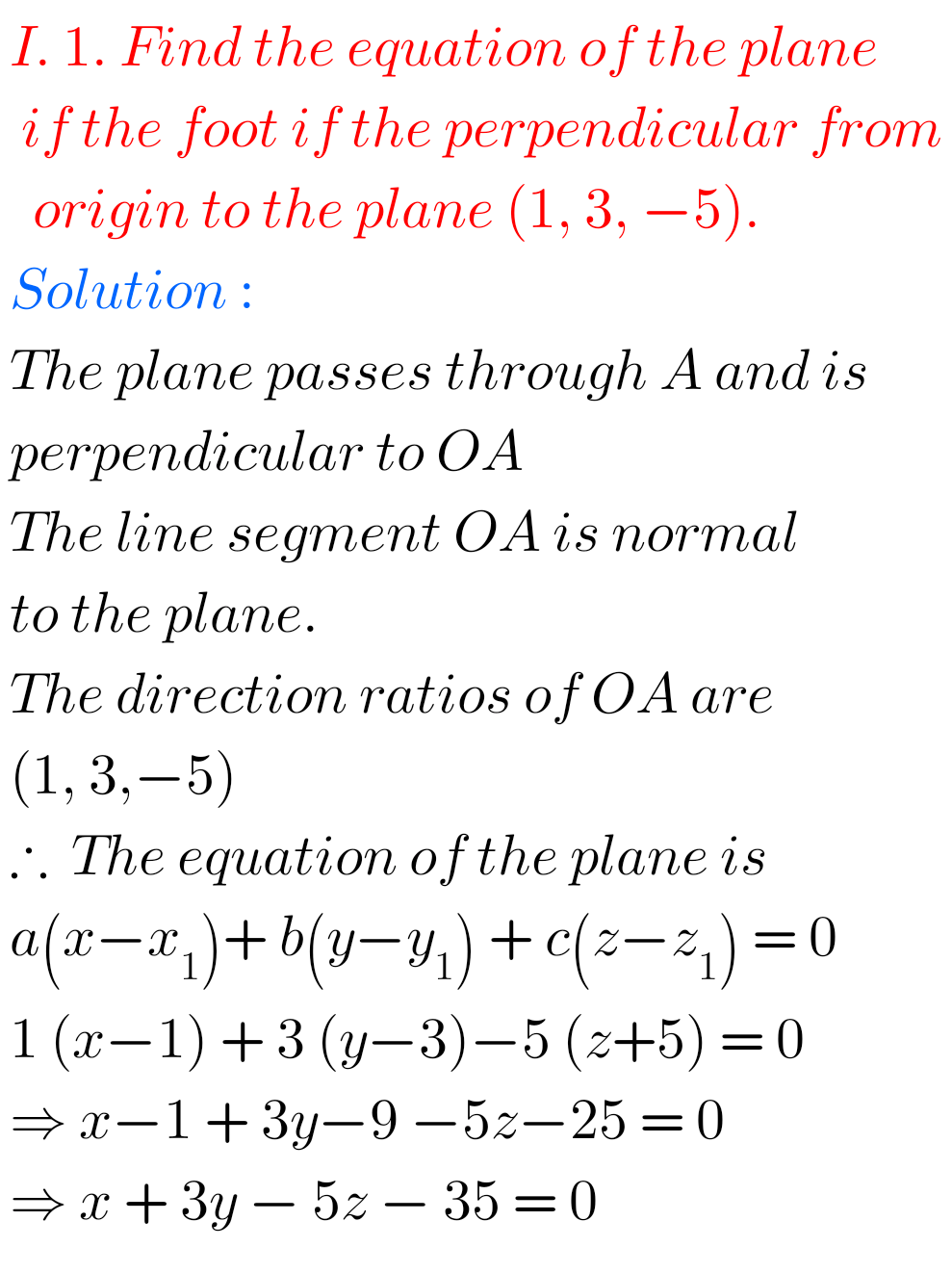The Plane Chapter 7 Exercise 7(a) Questions number I –  2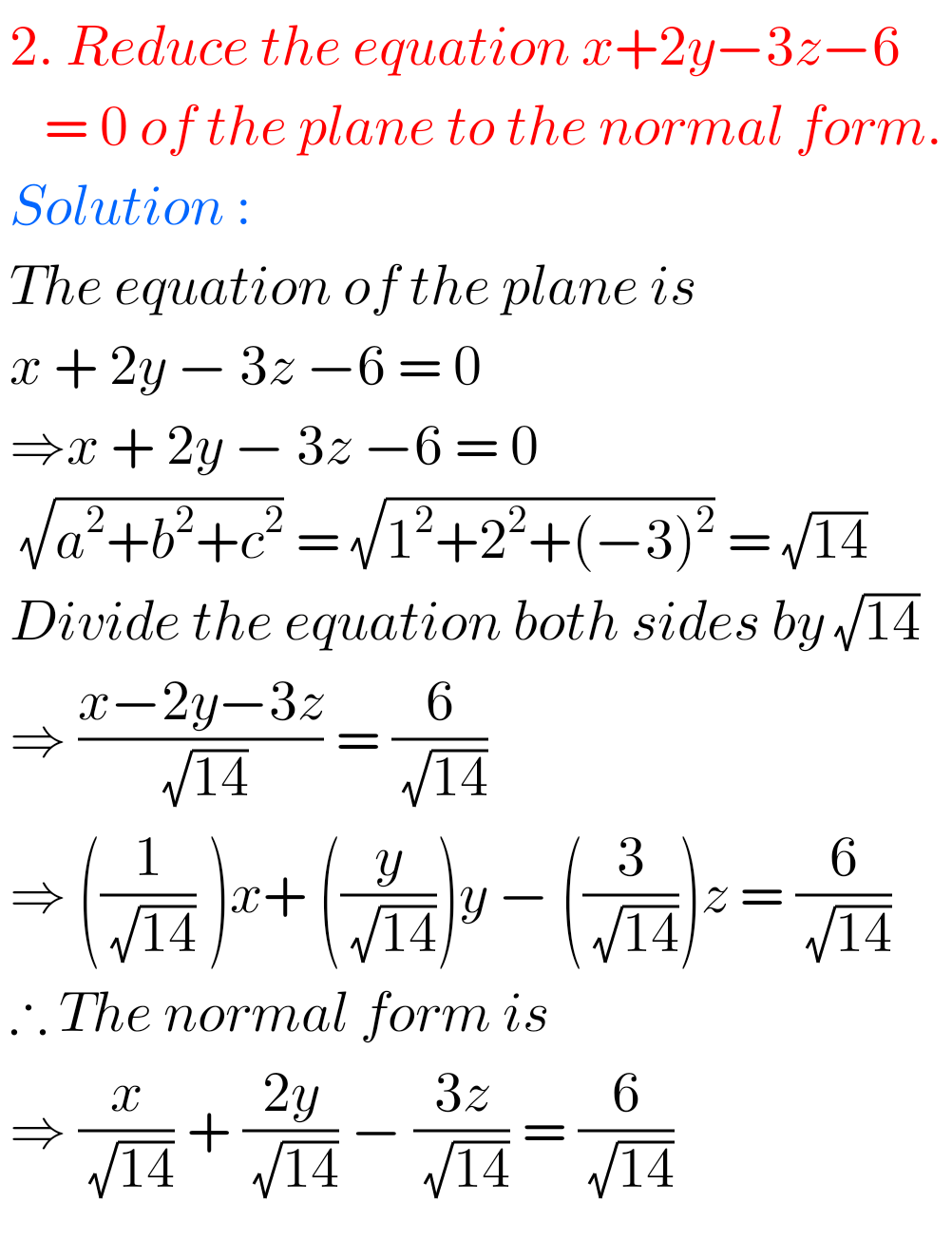The Plane Chapter 7 Exercise 7(a) Questions number I –  3The Plane Chapter 7 Exercise 7(a) Questions number I –  4The Plane Chapter 7 Exercise 7(a) Questions number I –  5The Plane Chapter 7 Exercise 7(a) Questions number I –  6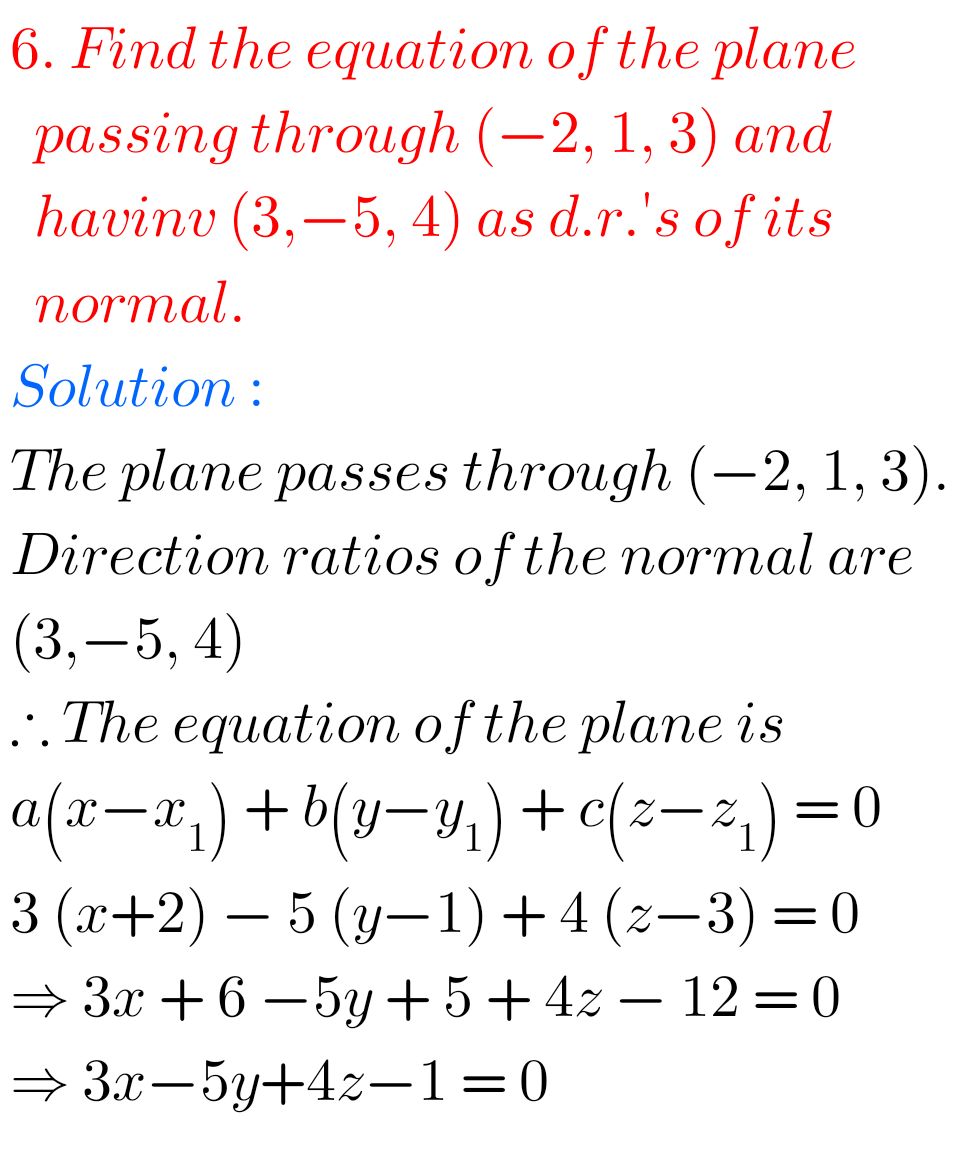The Plane Chapter 7 Exercise 7(a) Questions number I –  7The Plane Chapter 7 Exercise 7(a) Questions number I –  8## The Plane Solutions Inter Maths 1b

The Plane Chapter 7 Exercise 7(a) Questions number II –  1The Plane Chapter 7 Exercise 7(a) Questions number II –  2The Plane Chapter 7 Exercise 7(a) Questions number II –  3The Plane Chapter 7 Exercise 7(a) Questions number II –  4The Plane Chapter 7 Exercise 7(a) Questions number II –  5The Plane Chapter 7 Exercise 7(a) Questions number II –  6The Plane Chapter 7 Exercise 7(a) Questions number II –  7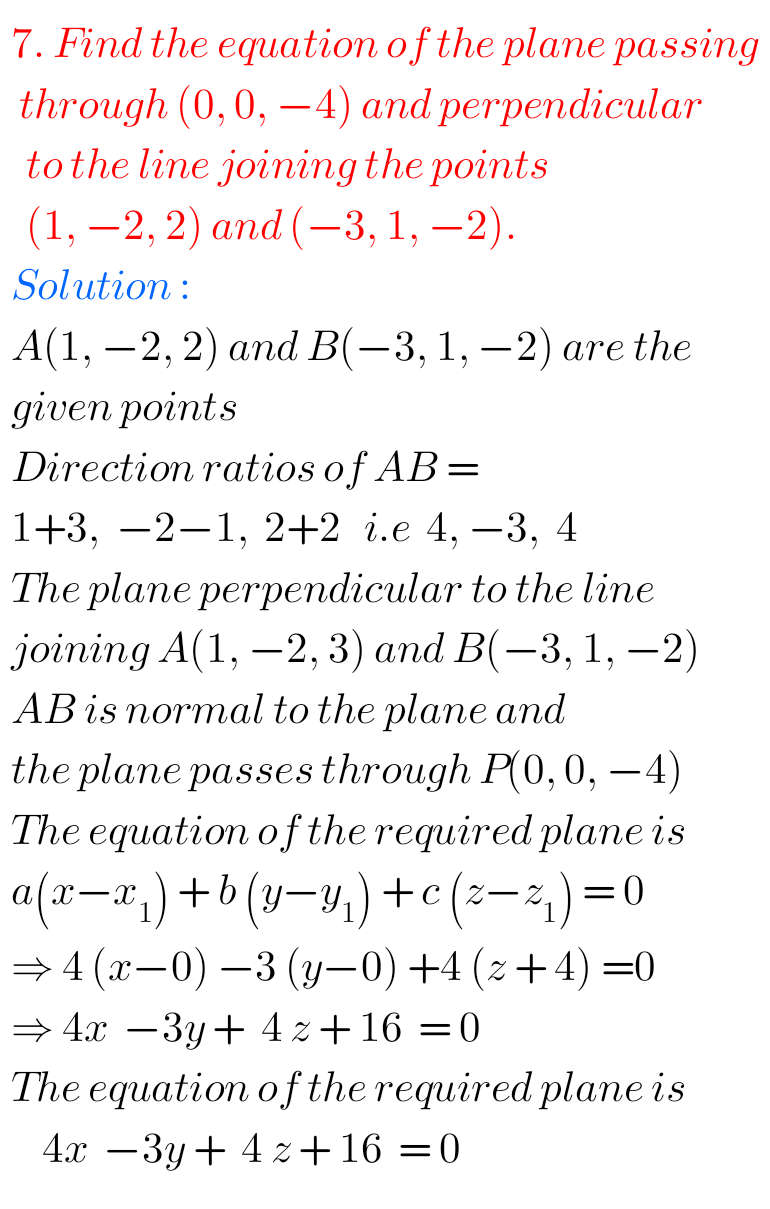The Plane Chapter 7 Exercise 7(a) Questions number II –  8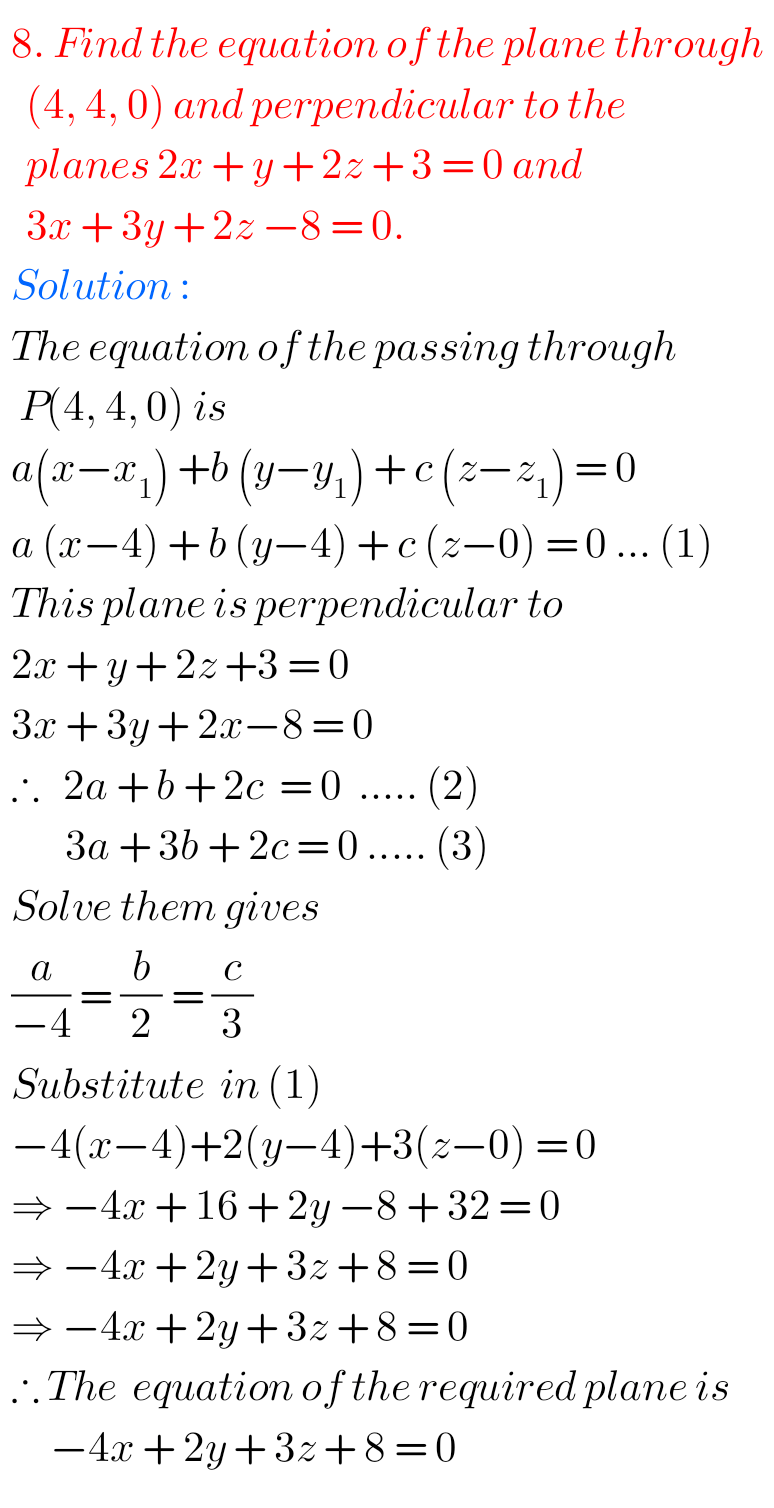### Solutions for The Plane Chapter 7 Exercise 7(a)

The Plane Chapter 7 Exercise 7(a) Questions number III – 1The Plane Chapter 7 Exercise 7(a) Questions number III – 2The Plane Chapter 7 Exercise 7(a) Questions number III – 3The Plane Chapter 7 Exercise 7(a) Questions number III – 4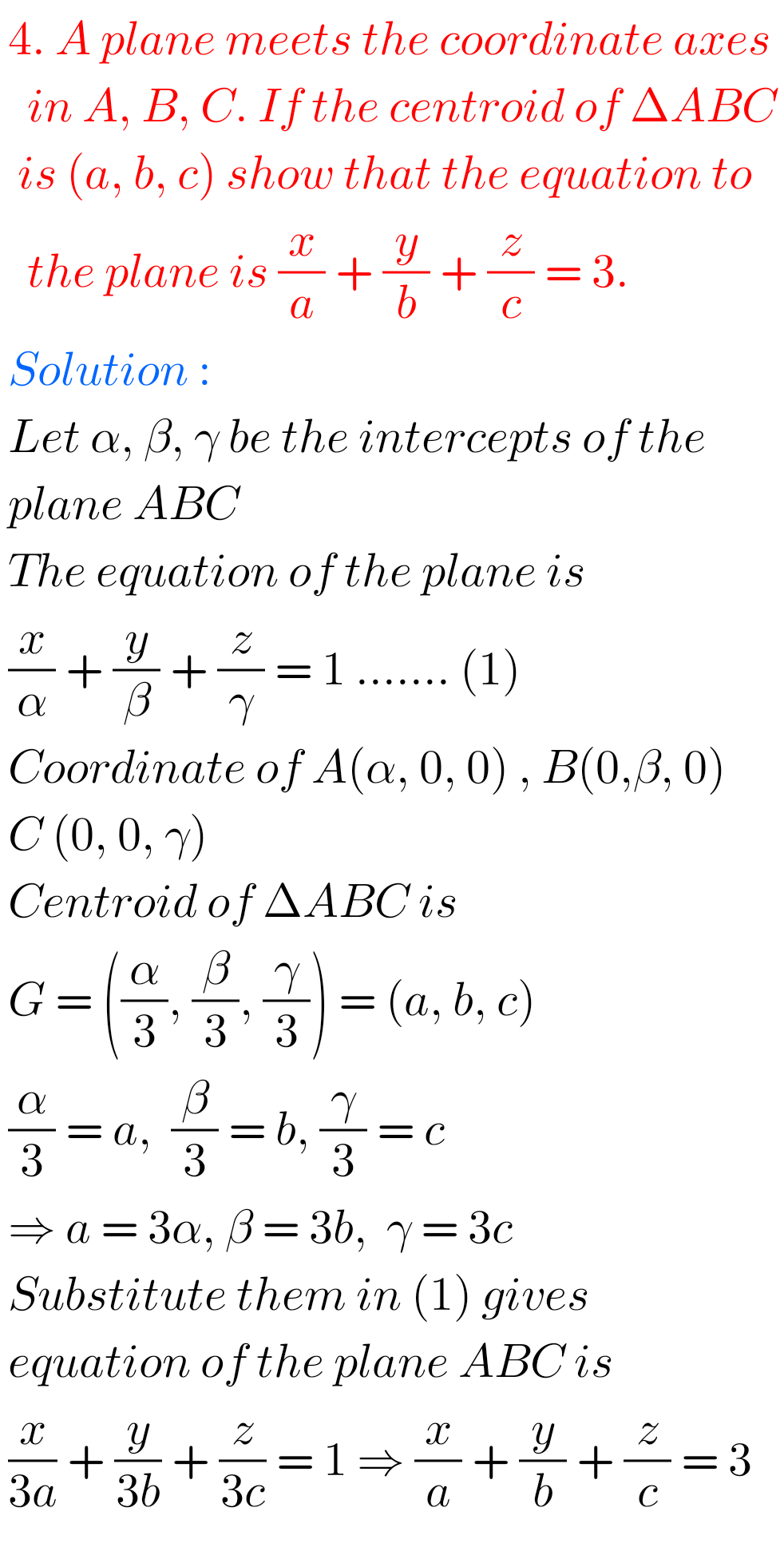The Plane Chapter 7 Exercise 7(a) Questions number III – 5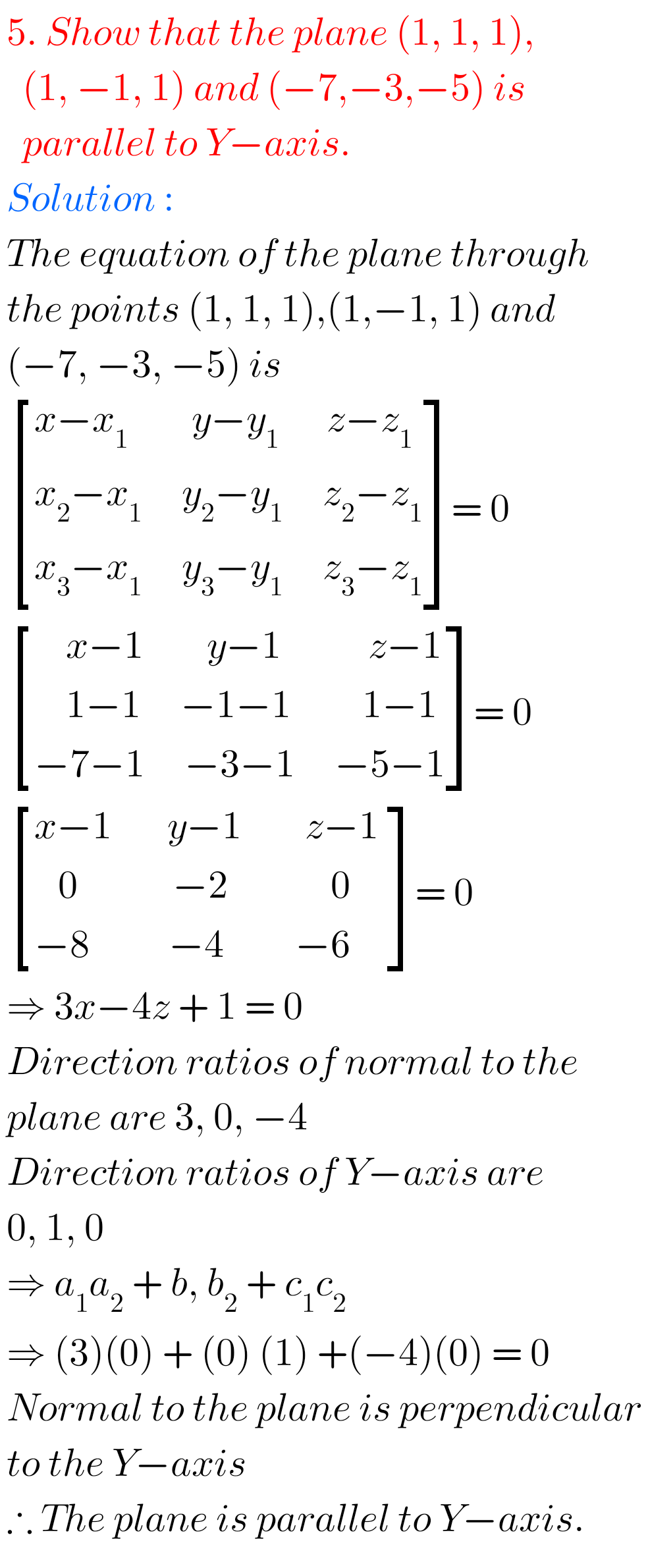The Plane Chapter 7 Exercise 7(a) Questions number III – 6M

Note : Observe the solutions and try them in your own method.

SSC Maths Solutions class 10 textbook

Inter Maths 1A textbook solutions

Inter Maths 1IA textbook solutions

Inter Maths IIB textbook solutions